# Block and Drilling Line Calculation

This article will focus on block and drilling line calculations as block efficiency, drilling power input/output, etc. Additionally, there are some examples which will help you understand how the formulas work.Block and drilling line efficiency formula is described below;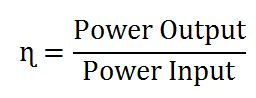Power Output (Po) = Fh x Vtb

Power Input (Pi) = Ff x VfWhere;

Fh is the hook load in lb.

Vtb is the velocity of the travelling block in feet per minute (fpm).

Ff is the fast line load in lb.

Vf is the velocity of the fast line in feet per minute (fpm).

The relationship between the fast line speed and the travelling block speed is listed below;

Vf = n x Vtb

Where;

n is number of lines strung between the travelling block and the crown block.

We can describe the hoisting efficiency into the following form.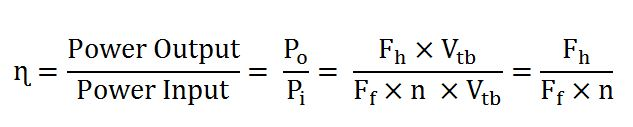Example#1: The maximum anticipated hook load is 500,000 and the travelling block velocity is 100 ft/minute. Hoisting efficiency is 85% and the drawworks efficiency is 75%. Based on the given information, what is the required horsepower for the drawworks for this situation?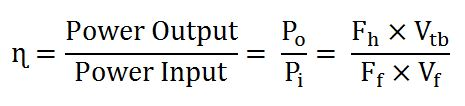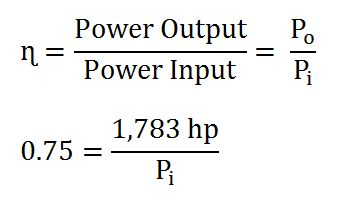Pi = 58,832,529 ft-lb/min

1 horsepower = 33,000 ft-lb/min

Pi = 58,832,529 ÷ 33,000 = 1,783 hp

We need to look at the drawworks. The drawworks output is the hoisting system output therefore we can figure out the horse power of the drawworks.

Pi = 2,377 hp

You need input horsepower of the drawworks of 2,377 hp.

Example#2: The string will be pulled out from 15,000 ft. The rig information is listed below;

Drilling lines: 12 lines

Hoisting efficiency: 70%

Drawworks: 1,200 hp

Drawworks efficiency: 80%

Expected hookload at 15,000 ft: 350,000 lb

What is the speed at which the first stand (90 ft stand) can be pulled out of hole based on the drawworks technical limit?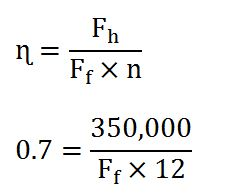Ff = 41,667 lb

Fast line velocity (Vf) = Power Input (Pi) ÷ Fast line Load (Ff)

Power Input (Pi) comes from the drawworks and we can determine it from the drawworks efficiency.

Power Input (Pi) = 0.8 x 1,200 = 960 hp

1 horsepower = 33,000 ft-lb/min

Power Input (Pi) = 960 x 33,000 = 31,680,000 ft-lb/min

Fast line velocity (Vf) = 31,680,000 ÷ 41,667 = 760 fpm

Travelling block velocity can be calculated by the following equation.

Vf = n x Vtb

Vtb = Vf ÷ n

Vtb = 760÷ 12 = 63.3 fpm

The travelling block speed = 90 ÷ 63.3 = 1.42 minute.

The pulling speed for the first stand is 1.42 minutes.

Ref books: Lapeyrouse, N.J., 2002. Formulas and calculations for drilling, production and workover, Boston: Gulf Professional publishing.

Bourgoyne, A.J.T., Chenevert , M.E. & Millheim, K.K., 1986. SPE Textbook Series, Volume 2: Applied Drilling Engineering, Society of Petroleum Engineers.

Mitchell, R.F., Miska, S. & Aadny, B.S., 2011. Fundamentals of drilling engineering, Richardson, TX: Society of Petroleum Engineers.

Share the joy
Tagged , , . Bookmark the permalink.Working in the oil field and loving to share knowledge.

### 4 Responses to Block and Drilling Line Calculation

1.Rahul says:

There is a typing mistake. Instead of Power input it says Power Output.
Power Output (Pi) = Ff x Vf

Third line from the picture.

•DrillingFormulas.Com says:

Rahul,

The typo mistake was corrected.

Regards,
Shyne

2.Prashant says:

In Example 1 please check the block velocity. It should be 100 fpm according the solution.

•DrillingFormulas.Com says:

Prashant,

Thanks for informing us. The typo error is fixed.

Regards,
Shyne.

This site uses Akismet to reduce spam. Learn how your comment data is processed.